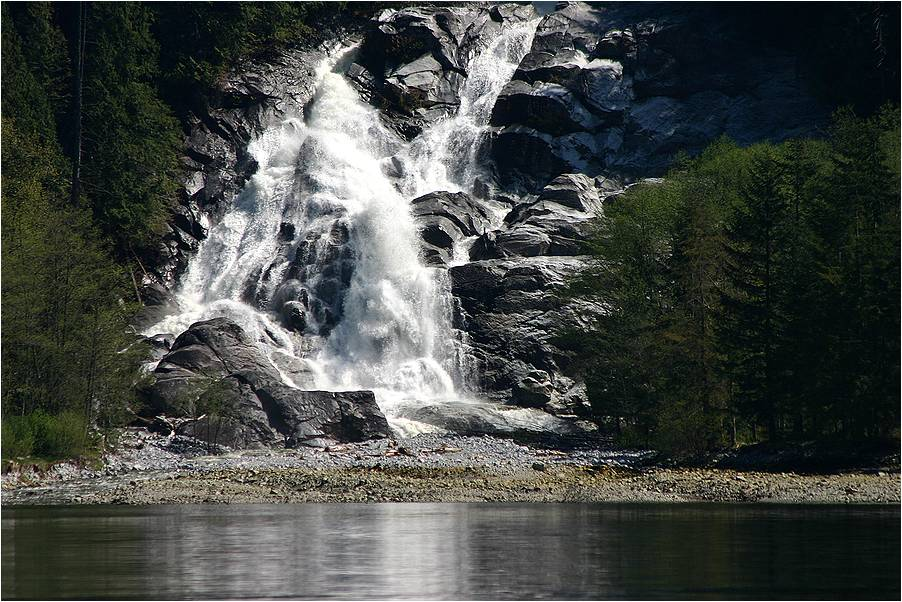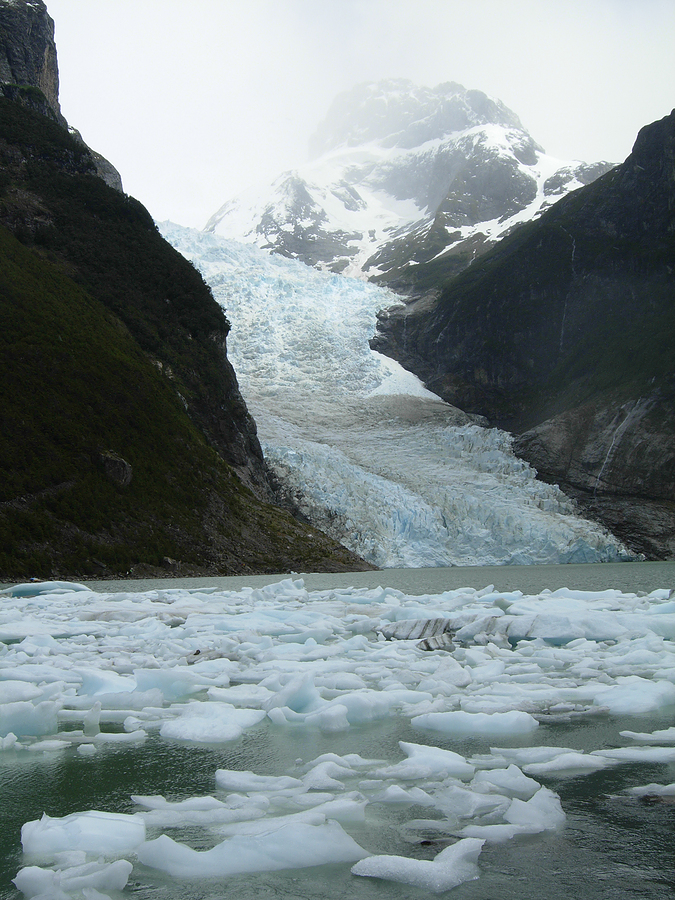Gravity

Gravity is physical force by which objects attract or pull toward each other. Gravitational force occurs between all objects that have mass. All matter has mass, which is the amount of matter an object contains. The strength of the gravitational force between two objects depends on two factors: the mass of each of the objects and the distance between the centers of the two objects. The more mass an object has, the greater the pull of its gravitational force on another object. For example, Earth has greater gravitational force than basketball. In addition, the closer the distance between two objects, the greater the gravitational force between them. The Sun’pull on Earth is stronger than Alpha Centauri’pull on Earth. Even though Alpha Centauri is 1.times the mass of the Sun, it is 4.light-years away from Earth instead of light-minutes from Earth (like the Sun).The Sun has more gravitational pull than a basketball does because it has more mass. Mass and distance are key factors in determining strength of gravitational pull on an object.

Weight and mass are two different measurements. Whereas mass is the amount of matter contained in an object, weight is measurement of the gravitational force exerted on given object that is at rest on larger body’surface. When you step on scale, you are measuring the force of Earth’gravity pulling downward on your mass. The weight of an object depends on the strength of the gravitational force acting on it. Although an object has the same mass no matter where it is located, an object weighs less where there is less gravity. The Moon has only 1/81 as much mass as Earth. Therefore, person weighs less on the Moon than on Earth. satellite such as the International Space Station is being pulled toward Earth, but it is moving so fast around us that it “misses” Earth as it falls. The orbital motion exactly counterbalances Earth’gravitational attraction, so everything is weightless in the International Space Station. Gravity is what holds the planets in our solar system in orbit around the Sun. Without the Sun’gravity, the planets would travel in straight line out into deep space.A person on the surface of the Moon only weighs 1/6 of what that same person weighs on the surface of Earth.

The gravitational force on Earth continually pulls everything straight down toward the planet’center. As result, ball tossed in the air falls to the ground. Snowflakes produced in the clouds float down from the sky, and leaves fall from trees. Rivers in the mountains flow downward toward oceans, and glaciers tumble down from mountains to form icebergs in oceans and lakes. The pull of Earth’gravity downward is why rockets flying into orbit require engine boosters to push them up; they must overcome the force of Earth’gravity.Earth’s gravity pulls everything down toward Earth’s center.

Everything on Earth experiences other types of forces in addition to gravity, among which are friction, elasticity, pushing, and pulling. Suppose you were to drop two objects with different masses at the same time from the same height. Ignoring all the other forces, especially air friction, Earth’gravity causes the two objects to fall (accelerate) downward at the same rate. They will both reach the Earth’surface at the same time. If you went into vacuum chamber and dropped feather and bowling ball from the top of three-story ladder, they would fall straight down toward the center of Earth and would hit the floor at the exact same time.If other forces are taken out of the equation, Earth’s gravity causes all objects to fall at the same rate.

Fifteenth-century physicist Isaac Newton was inspired by his observations of objects falling in nature and used them as the basis of his theory of universal gravitation. Newton proposed that the force of gravity attracts all matter to all other matter and that force can be calculated knowing the masses of the two attracting objects and the distance between their centers. The less massive or the farther apart two objects are, the less the gravitational force between them is. We generally think about gravity on the scale of planets. It is important to note, however, that all objects have gravity acting between them, even between person and an apple. This gravitational interaction is much, much weaker than between the person and Earth, so it seems negligible and is not detectable in everyday life.Isaac Newton was instrumental in discovering laws about gravity.